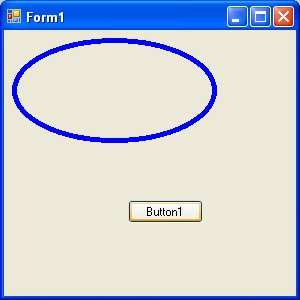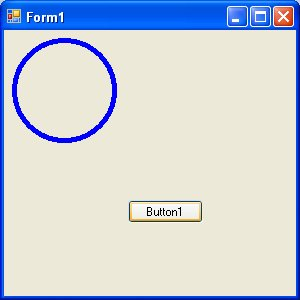# Lesson 23 : Drawing Ellipse and Circle

In this lesson, we will learn how to draw ellipse and circle using the DrawEllipse method.

### 23.1 Drawing Ellipse with the DrawEllipse Method

The basic structure of most shapes is a rectangle, an ellipse is no exception. Ellipse is an oval shape that is bounded by a rectangle, as shown in Figure 23.1#### Figure 23.1

Therefore, we need to create a Rectangle object before we can draw an ellipse. This rectangle serves as a bounding rectangle for the ellipse. We still need to use the DrawEllipse method to complete the job.

On the other hand, we can also draw an ellipse with the DrawEllipse method without first creating a rectangle. We shall illustrate both methods.

In the first method, let's say you have created a rectangle object known as myRectangle and a pen object as myPen, then you can draw an ellipse using the following statement:

```myGraphics.DrawEllipse(myPen, myRectangle)
```

* Assume you have also already created the Graphics object myGraphics.

The following is an example of the full code:

#### Example 23.1(a)

```Dim myPen As Pen
myPen = New Pen(Drawing.Color.Blue, 5)
Dim myGraphics As Graphics = Me.CreateGraphics
Dim myRectangle As New Rectangle
myRectangle.X = 10
myRectangle.Y = 10
myRectangle.Width = 200
myRectangle.Height = 100
myGraphics.DrawEllipse(myPen, myRectangle)
```

The output image is shown in Figure 23.2#### Figure 23.2

The second method is using the DrawEllipse method without creating a rectangle object. Of course, you still have to create the Graphics and the Pen objects. The syntax is:

```myGraphics.DrawEllipse(myPen, X,Y,Width, Height)
```

Where (X, Y) are the coordinates of the upper-left corner of the bounding rectangle, width is the width of the ellipse and height is the height of the ellipse.

The following is an example of the full code:

#### Example 23.1(b)

```Dim myPen As Pen
myPen = New Pen(Drawing.Color.Blue, 5)
Dim myGraphics As Graphics = Me.CreateGraphics
myGraphics.DrawEllipse(myPen, 10, 10, 200, 100)
```

### 23.2 Drawing a Circle

After you have learned how to draw an ellipse, drawing a circle becomes very simple. We use exactly the same methods used in the preceding section but modify the width and height so that they are of the same values.

The following examples draw the same circle.

#### Example 23.2(a)

```Dim myPen As Pen
myPen = New Pen(Drawing.Color.Blue, 5)
Dim myGraphics As Graphics = Me.CreateGraphics
Dim myRectangle As New Rectangle
myRectangle.X = 10
myRectangle.Y = 10
myRectangle.Width = 100
myRectangle.Height = 100
myGraphics.DrawEllipse(myPen, myRectangle)
```

#### Example 23.2(b)

```Dim myPen As Pen
myPen = New Pen(Drawing.Color.Blue, 5)
Dim myGraphics As Graphics = Me.CreateGraphics
myGraphics.DrawEllipse(myPen, 10, 10, 100, 100)
```

The output image is shown in Figure 23.3.